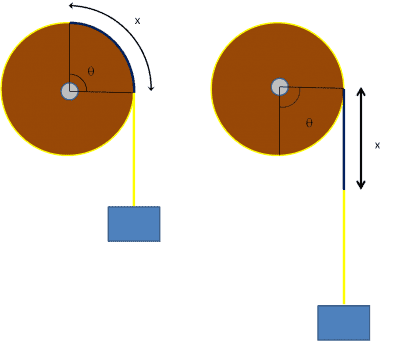# Angular acceleration on a pulley

df102015

## Homework Statement

[/B]
A mass of 6.1 kg tied to a string is wrapped around a disk as shown. If the disk has a mass of 8.2 kg and a radius of 2.3 m, how fast will the disk be rotating when the weight has fallen 7.4 m and was released from rest?Θ = S/R = x/R
Θ = 0.5 α t^2
τ = I α
τ = T R
T = mg - ma
I = 0.5 M R^2

## The Attempt at a Solution

These are the equations that my teacher gave me. So far, when i combined them i get this...

α = τ/I = T R / 0.5 M R^2 = (mg - ma) R / 0.5 M R^2 = (mg - ma) / 0.5 M R

Which, when numbers are substituted in becomes...

(6.1 x 9.81 - 6.1a) / 0.5 x 8.2 x 2.3 = α

but i still do not have acceleration (a) and the equation
a = Δv / t
gives me two other things that i do not have... change in velocity and time. And the equations using theta that he gave me seem almost useless because the value of theta is not given, although it looks as if it is 90 degrees, but i could be wrong. When i calculate theta using x/R i got 3.2174 which i assume is in pi radians, but could just be wrong altogether. Can somebody please just point me in the right direction, help me with some different equations if they are needed, it is the last problem that i need to finish on the homework. Also i believe my teacher may have mentioned there is a way to solve by using energy equations, if that is easier then please help using that. Thanks so much!

Homework Helper
Gold Member
Energy is a nice way to solve it. But you've already done most of the work in using the method of forces and torques. All you need is another relation between α and a. I bet you've covered that relation. It's similar to the equation s = Rθ.

df102015
is the equation i am looking for α = a/R ?

Homework Helper
Gold Member
Yes, that's it.

•df102015
df102015
Yes, that's it.
Thanks so much!!!! You have no idea how much i appreciate it!

Sahil Kukreja
Another way to solve is by conservation of energy:-

loss in G.P.E. of box = gain in K.E. of box + gain in K.E. of Disc.

## mgh=\frac{1}{2} mv^2 + \frac{1}{2} I\omega^2\ ##
## \omega\ ## is clockwise
and for no slipping ## v=r \omega\ ##